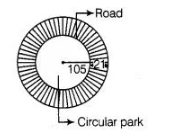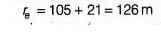# A circular park is surrounded by a road 21 m wide.

Question:

A circular park is surrounded by a road 21 m wide. If the radius of the  park is 105 m, then find the area of the road.

Solution:

Given that, a circuiar park is surrounded by a road.

Width of the road $=21 \mathrm{~m}$

Radius of the park $(r)=105 \mathrm{~m}$$\therefore$ Radius of whole circular portion (park + road),Now, area of road = Area of whole circular portion

- Area of circular park

$=\pi r_{8}^{2}-\pi r_{i}^{2} \quad\left[\therefore\right.$ area of circle $\left.=\pi r^{2}\right]$

$=\pi\left(t_{e}^{2}-r_{i}^{2}\right)$

$=\pi\left\{\left(126^{2}-(105)^{2}\right\}\right.$

$=\frac{22}{7} \times(126+105)(126-105)$

$=\frac{22}{7} \times 231 \times 21 \quad\left[\because\left(a^{2}-b^{2}\right)=(a-b)(a+b)\right]$

$=66 \times 231$

$=15246 \mathrm{~cm}^{2}$

Hence, the required area of the road is $15246 \mathrm{~cm}^{2}$.